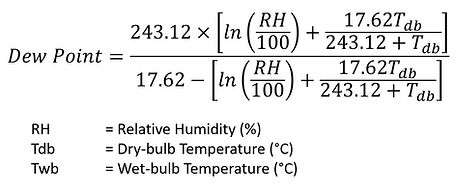Simple Psychrometric Calculator

Psychrometric Equations are hard to represent in simple mathematical models with high degree of accuracies.

This calculator referred to various mathematical models from a number of websites that uses relatively simpler, spreadsheet-compatible mathematical equations to estimate values of variables: Relative Humidity (RH), Dry Bulb Temperature, Tdb, Wet Bulb Temperature, Twb, and Dew Point Temperature, Tdp, to a limited degree of accuracy, and within a specific range of temperature input.

In view of the relative simplicity of the mathematical model in this calculator, equations that resemble higher accuracies or effect from other factors such as elevation, shall be referred from ASHRAE or other more advanced psychrometric calculator sites.

Refer to the other page Advanced Psychrometric Calculator which mathematically approximates values from the psychrometric chart with acceptable degree of accuracy.

Estimating Enthalpy (From Tdb and RH)

Enthalpy, h of moist air can be estimated from dry-bulb temperature, Tdb and Relative Humidity (RH%) based on the polynomial function below:

h = 0.24*TDB+(0.6219)*(0.01*(0.000000007401234*TDB^4 - 0.000000493526794*TDB^3 + 0.000071281097208*TDB^2 - 0.000489806163078*TDB + 0.039762055806989)*RH)/(14.7-(0.01*(0.000000007401234*TDB^4 - 0.000000493526794*TDB^3 + 0.000071281097208*TDB^2 - 0.000489806163078*TDB + 0.039762055806989)*RH))*(1061.2+0.444*TDB)

Where

h = Enthalpy (btu/lb)

TDB = Dry-bulb Temperature (°F)

RH = Relative Humidity (%)

This model represents the psychrometric curve up to 99% accuracy for dry-bulb temperature from 25°F to 100°F.

Reference:

Estimating Wet-bulb Temperature Calculator (From Tdb and RH)

On the other hand, the value of Wet-bulb Temperature, Twb can be determined from Relative Humidity (RH%) and Dry-bulb Temperature, Tdb.

TWB = (((TDB-32)*(5/9)*ATAN(0.151977*((RH+8.313659)^(1/2))))+(ATAN((TDB-32)*(5/9)+RH))-(ATAN(RH-1.676331))+(0.00391838*((RH)^(3/2))*ATAN(0.023101*RH))-4.686035)*(9/5)+32

Where

TWB = Wet-bulb Temperature (°F)

TDB = Dry-bulb Temperature (°F)

RH = Relative Humidity (%)

This model represents the psychrometric curve up to 97% accuracy for dry-bulb temperature from 45°F to 150°F.

Estimating RH (From Tdb and Twb)

Relative humidity (RH) is the ratio of the actual, partial pressure of water vapor to the equilibrium, saturated vapor pressure of water at a given temperature. The value of Relative Humidity, RH, can be determined from Dry-bulb Temperature, Tdb, and Wet-bulb Temperature, Twb.

es = 6.112*EXP((17.67*TDB)/(TDB+243.5))

ew = 6.112*EXP((17.67*TWB)/(TWB+243.5))

e = ew - Pstd*(TDB-TWB)*0.00066*(1+0.00115*TWB)

RH = e/es*100

Where

es           = Saturated Vapour Pressure (mbar)

ew          = Vapour Pressure related to Wet-bulb Temperature (mbar)

e             = Actual Vapour Pressure (mbar)

Pstd       = Standard Atmospheric Pressure (mbar), 1013.23876 mbar

TWB      = Wet-bulb Temperature (°C)

TDB        = Dry-bulb Temperature (°C)

RH          = Relative Humidity (%)

Substituting e & es into the RH equation,

RH = ((6.112*EXP((17.67*TWB)/(TWB+243.5))) - Pstd*(TDB-TWB)*0.00066*(1+0.00115*TWB))/ ( 6.112*EXP((17.67*TDB)/(TDB+243.5)))*100Apart from using a formula, RH can be determined by referring to a dry-bulb temperature and the difference between the dry-bulb temperature and a wet-bulb temperature in the chart belowThis model represents the psychrometric curve up to 97% accuracy for dry-bulb temperature from 40°F to 275°F.

Estimating Dew Point Temperature (From Tdb and RH)

Dew point is the temperature at which condensation of water vapour occurs. Dew Point can be calculated using the Magnus formula, which is expressed in terms of dry-bulb temperature, Tdb and Relative Humidity, RH.

RH = (243.12*(LN(RH/100)+((17.62*TDB)/(243.12+TDB))))/(17.62-(LN(RH/100)+((17.62*TDB) /(243.12+TDB))))This model represents the psychrometric curve up to 96% accuracy for dry-bulb temperature from 40°F to 475°F & RH > 35%.